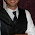Tuesday, April 26, 2011

Automatically Save Your Plots to a Folder

Suppose you're working on a problem that involves a loop for calculations. At each iteration inside the loop, you want to construct a plot. Not only do you want to see the plot, but you would like to save each plot for a presentation, report or paper. Furthermore, the loop goes on for a while (say through the 26-letters of the alphabet).

The last thing you want to do in this situation is: (1) produce each plot one-by-one, (2) right click on each singly-produced plot to save, (3) give the plot a unique name, and (4) repeat. You'll spend too much of your time saving plots and not enough time thinking about whether they are the right plots. Just imagine, what if something went wrong and you need to produce the whole set of plots again?

RStudio has a nice feature in that it saves all of your plots in the plotting pane. It's no problem if you just produce the plot inside your dreaded loop in RStudio because it keeps all of your plots in the pane. Even with RStudio, if you produce the plots inside the loop, you still need to save each one individually. This isn't ideal. If you don't believe me, imagine that you have 1000 plots instead of 26. This manual-saving method becomes impractical quickly.

How then can you automatically save plots to a folder without spending too much time? That's today's task. I'll start by describing several building-block commands and then at the end I'll put together a loop that does it all (by way of contrived example).

The first command you need to know is jpeg() (Alternatively, bmp(), png() or tiff(), depending on your file-type preferences) paired with dev.off(). For our purposes, jpeg() takes a path argument that allows us to save (at the location of our choosing via the path) output to a plotting window. For example, this code will save the next plotting object to a jpeg file called myplot.jpg located at "C://R//SAVEHERE"

jpeg(file = "C://R//SAVEHERE//myplot.jpg")

After running the plotting object, you need to be sure to turn off the plotting device you created (with the jpeg() command). To save a scatter plot of the vectors x versus y to the location described above, run these three lines:

jpeg(file = "C://R//SAVEHERE//myplot.jpg")
plot(x,y)
dev.off()

This code is a good building block for automatically saving to a folder inside a loop, but we still need to know how to dynamically create file names at which to save our plots. Suppose we have a vector that gives us a list of identifiers called names. Presumably, these identifiers mean something in your setting. For our working example, names is a list of letters from A to Z (we are trying to produce a separate scatter plot for each letter from A to Z).

We can use the file.path() and paste() commands to help us out here. I have found paste() to be incredibly useful for other applications as well. For example,

paste("myplot_", i, ".jpg", sep="")

produces the character string myplot_50.jpg when i = 50 and myplot_51.jpg when i=51. If i changes for each iteration in the loop, this paste() command will create a unique file name at each iteration.

We can get fancier with our pasting. If we have a vector of names, we can extract the ith name from the names vector with the paste command:

paste("myplot_", names[i], ".jpg", sep="")

The file.path() function helps us in a different way. It is a special paste function that helps us construct file paths really easily. For example,

mypath=file.path("C:", "R", "SAVEHERE", filename)

returns "C://R//SAVEHERE//filename" and stores it in an object called mypath. Replace filename with the paste command and we have a way to automatically generate file names in a folder called SAVEHERE.

Now, you have the tools to understand my example code of how to automatically generate and save plots to a folder. Make sure the folder exists before saving, but subject to that constraint, this procedure may make your life easier.

1.Great advice. I use this exact strategy myself. In fact, it can be very useful in creating animations as well (see the bottom of the post):

http://princeofslides.blogspot.com/2011/03/umpire-strike-zones.html

2.I always use pdf() command for plot output. How's the resolution of the jpeg or png output from R? I gave up on them because these bitmap-based-images are usually too blur to use.

3.@Everett

That's a good point that .jpg files have worse resolution. If you rerun the post with pdf() instead of jpeg(), it is sharper. Once I port them into a LyX document, I find that the resolution is fine for my purposes, but I can understand demanding sharper resolution.

4.Also, I spotted and fixed a weird quirk in my code for the plot output (giving a different title for each plot).

Originally, I instinctively used expression("my title is ", names[i]) when I really meant to use paste("my title is ", names[i]).

5.Use PNG instead of JPEG for your scientific plots. See http://goo.gl/cUHQ for why.

6.Nice illustration, Anonymous. Thanks for the tip.

7.Instead of png() ... dev.off() etc, you can use devEval() of the R.utils package to do the following:

# Change the output figure path (from the default ./figures/):
options("devEval/args/path"=file.path("C:","R","SAVEHERE"));

# Plot to a PNG file, e.g. myplot,A.png
main <- sprintf("my title is %s", names[i]);
devEval("png", name="myplot", tags=names[i], {
plot(x,y, main=main);
})

# Plot to a PDF file, e.g. myplot,A.pdf
devEval("pdf", name="myplot", tags=names[i], {
plot(x,y, main=main);
})

Note that you do not have to worry about filename extensions - they are added automatically.
You can also easily change the aspect ratio using argument 'aspectRatio' and so on.

8.Is it possible that in line 17 it should say "jpeg" instead of "jpg"?

I get an error message otherwise.